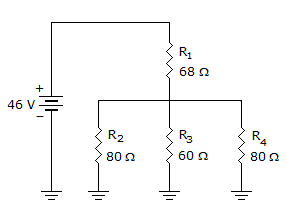# Electronics - Series-Parallel Circuits - Discussion

### Discussion :: Series-Parallel Circuits - General Questions (Q.No.11)

11.What is the total resistance of the given circuit?

 [A]. 92[B]. 288[C]. 17.7[D]. 128Explanation:

No answer description available for this question.

 Shilpa Shankar said: (Jun 24, 2011) r2*r4 /r2+r4 = 40 40*r3 /40+r3 =24 Therefore finally series of resultant of those paralle combination with 68 ohm resistance equals to 92 ohms.

 Madhusudan said: (Jul 23, 2011) 80||60||80=24 and so tot re=24+68=92

 Oosaravelli said: (Feb 4, 2012) 80||60||80 = 24 how ?

 Kushal Soni said: (Jun 26, 2012) 1/Rt= (1/80)+(1/60)+(1/80) = 1/24 Rt= 24

 Paresh Khatri said: (Sep 16, 2017) Good explanation @Shilpa. @Kushal. According to your explanation, we can not get this answer (24).

 Pari Khan said: (Oct 7, 2017) 1/60+1/80+1/80=24. Rt=24+68=92.

 Jerfree Pfundira said: (Jan 13, 2020) 68+1÷(1/80+1/60+1/80) = 68+24 = 92.